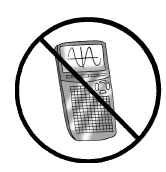### Home > CALC3RD > Chapter Ch9 > Lesson 9.4.1 > Problem9-112

9-112.

No calculator! Evaluate the following limits.

1. $\lim\limits _ { x \rightarrow \infty } \frac { 3 x ^ { 5 } - 2 x ^ { 2 } + 1 } { 2 x ^ { 6 } + 4 x - 1 }$

What is the dominant term in the numerator? What is the dominant term in the denominator? How do these terms compare?

1. $\lim\limits _ { x \rightarrow - \infty } e ^ { - 2 x }$

Graph $y = e^{–2x}$. What happens to the graph as $x → -∞$?

1. $\lim\limits _ { x \rightarrow 0 } \frac { \operatorname { cot } ( x ) } { \operatorname { ln } ( x ) }$

l'Hôpital's Rule can be used here. Or, you can graph the function.

1. $\lim\limits _ { n \rightarrow \infty } ( \displaystyle\sum _ { k = 1 } ^ { n } ( \frac { 1 } { 3 } ) ^ { k } )$

This is the geometric series:

$\frac{1}{3}+\frac{1}{9}+\frac{1}{27}+...$